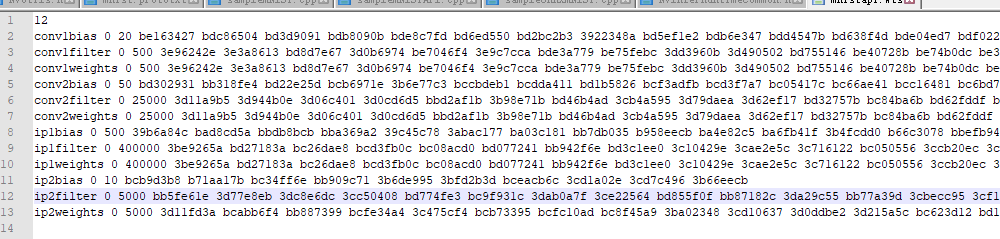# 2. 使用C++ API函数部署流程¶

//!
//! 简介： 使用caffe解析器创建MNIST网络并标记输出层
//!
//! 参数： 指向将用MNIST网络填充的网络指针
//!
//! 参数： 指向引擎生成器的生成器指针
//!
void SampleMNIST::constructNetwork(SampleUniquePtr<nvcaffeparser1::ICaffeParser>& parser, SampleUniquePtr<nvinfer1::INetworkDefinition>& network)
{
const nvcaffeparser1::IBlobNameToTensor* blobNameToTensor = parser->parse(
mParams.prototxtFileName.c_str(),
mParams.weightsFileName.c_str(),
*network,
nvinfer1::DataType::kFLOAT);

//输出Tensor标记
for (auto& s : mParams.outputTensorNames)
{
network->markOutput(*blobNameToTensor->find(s.c_str()));
}

// 在网络开头添加减均值操作
nvinfer1::Dims inputDims = network->getInput(0)->getDimensions();
// 读取均值文件的数据
mMeanBlob = SampleUniquePtr<nvcaffeparser1::IBinaryProtoBlob>(parser->parseBinaryProto(mParams.meanFileName.c_str()));
nvinfer1::Weights meanWeights{nvinfer1::DataType::kFLOAT, mMeanBlob->getData(), inputDims.d * inputDims.d};
// 数据的原始分布是[0,256]
// 减去均值之后是[-127,127]
// The preferred method is use scales computed based on a representative data set
// and apply each one individually based on the tensor. The range here is large enough for the
// network, but is chosen for example purposes only.
float maxMean = samplesCommon::getMaxValue(static_cast<const float*>(meanWeights.values), samplesCommon::volume(inputDims));

auto mean = network->addConstant(nvinfer1::Dims3(1, inputDims.d, inputDims.d), meanWeights);
mean->getOutput(0)->setDynamicRange(-maxMean, maxMean);
network->getInput(0)->setDynamicRange(-maxMean, maxMean);
// 执行减均值操作
auto meanSub = network->addElementWise(*network->getInput(0), *mean->getOutput(0), ElementWiseOperation::kSUB);
meanSub->getOutput(0)->setDynamicRange(-maxMean, maxMean);
network->getLayer(0)->setInput(0, *meanSub->getOutput(0));
// 执行缩放操作
samplesCommon::setAllTensorScales(network.get(), 127.0f, 127.0f);
// 最后的网络的输入就是[-1, 1]
}


## 2.1 创建网络¶

//!
//! 简介： 创建网络、配置生成器并创建网络引擎
//!
//! 细节： 此函数通过解析caffe模型创建MNIST网络，并构建用于运行MNIST（mEngine）的引擎
//!
//! 返回值： 如果引擎被创建成功，直接返回True
//!
bool SampleMNISTAPI::build()
{
//加载权重，*.wts文件

// 1. Create builder
//创建一个 IBuilder，传进gLogger参数是为了方便打印信息。
//builder 这个地方感觉像是使用了建造者模式。
auto builder = SampleUniquePtr<nvinfer1::IBuilder>(nvinfer1::createInferBuilder(gLogger.getTRTLogger()));
if (!builder)
{
return false;
}
//创建一个 network对象，但是这个network对象只是一个空架子，里面的属性还没有具体的数值。
auto network = SampleUniquePtr<nvinfer1::INetworkDefinition>(builder->createNetwork());
if (!network)
{
return false;
}
//创建一个配置文件解析对象
auto config = SampleUniquePtr<nvinfer1::IBuilderConfig>(builder->createBuilderConfig());
if (!config)
{
return false;
}
//利用C++ API创建网络
auto constructed = constructNetwork(builder, network, config);
if (!constructed)
{
return false;
}

assert(network->getNbInputs() == 1);
auto inputDims = network->getInput(0)->getDimensions();
assert(inputDims.nbDims == 3);

assert(network->getNbOutputs() == 1);
auto outputDims = network->getOutput(0)->getDimensions();
assert(outputDims.nbDims == 3);

return true;
}


## 2.2 为网络添加输入¶

// 为网络添加输入
mParams.inputTensorNames.c_str(), DataType::kFLOAT, Dims3{1, mParams.inputH, mParams.inputW});


enum class DataType : int
{
kFLOAT = 0, //!< FP32 format.
kHALF = 1,  //!< FP16 format.
kINT8 = 2,  //!< quantized INT8 format.
kINT32 = 3  //!< INT32 format.
};


## 2.3 添加各种层¶

• 添加一个Scale Layer。

// Create scale layer with default power/shift and specified scale parameter.
const float scaleParam = 0.0125f;
const Weights power{DataType::kFLOAT, nullptr, 0};
const Weights shift{DataType::kFLOAT, nullptr, 0};
const Weights scale{DataType::kFLOAT, &scaleParam, 1};
IScaleLayer* scale_1 = network->addScale(*data, ScaleMode::kUNIFORM, shift, scale, power);
assert(scale_1);


$f(x)=(shift+scale*x)^{power}$

power: 默认为1。
scale: 默认为1。
shift: 默认为0。


class Weights
{
public:
DataType type;      //!< The type of the weights.
const void* values; //!< The weight values, in a contiguous array.
int64_t count;      //!< The number of weights in the array.
};


Scale层是没有训练参数的，ReLU层，Pooling层都没有训练参数。而有训练参数的如卷积层，全连接层，在构造的时候则需要先加载权重文件。 - 添加一个20个通道的$5\times 5$卷积层的。

// Add convolution layer with 20 outputs and a 5x5 filter.
// 添加卷积层
IConvolutionLayer* conv1 = network->addConvolution(*scale_1->getOutput(0), 20, DimsHW{5, 5}, mWeightMap["conv1filter"], mWeightMap["conv1bias"]);
assert(conv1);
//设置步长
conv1->setStride(DimsHW{1, 1});


## 2.4 解析mnistapi.wts文件¶## 2.5 设定网络输出¶

// Add softmax layer to determine the probability.
assert(prob);
prob->getOutput(0)->setName(mParams.outputTensorNames.c_str());
network->markOutput(*prob->getOutput(0)


# 6. 同期文章¶